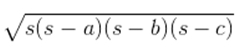Guru

# A triangle and a parallelogram have the same base and the same area. If the sides of the triangle are 26 cm, 28 cm and 30 cm, and the parallelogram stands on the base 28 cm, find the height of the parallelogram. Q.4

• 0

How i solve the question of class 9th ncert math of Heron’s Formula chapter of exercise 12.2  of question no 4. I think it is very important question of class 9th give me the tricky way for solving this question A triangle and a parallelogram have the same base and the same area. If the sides of the triangle are 26 cm, 28 cm and 30 cm, and the parallelogram stands on the base 28 cm, find the height of the parallelogram.

Share

1. It is given that the parallelogram and triangle have equal areas.

The sides of the triangle are given as 26 cm, 28 cm and 30 cm.

So, the perimeter = 26+28+30 = 84 cm

And its semi perimeter = 84/2 cm = 42 cm

Now, by using Heron’s formula, area of the triangle == √[42(42-26)(42-28)(42-30)] cm2

= √[42×16×14×12] cm2

= 336 cm2

Now, let the height of parallelogram be h.

As the area of parallelogram = area of the triangle,

28 cm× h = 336 cm2

∴ h = 336/28 cm

So, the height of the parallelogram is 12 cm.

• 0